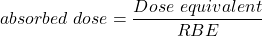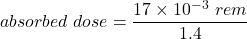## The yearly whole-body dose caused by radioactive 40K absorbed in our tissues is 17 mrem. Note: 40K also emits gamma rays, many of which leav

Question

The yearly whole-body dose caused by radioactive 40K absorbed in our tissues is 17 mrem. Note: 40K also emits gamma rays, many of which leave the body before being absorbed. Because fatty tissue has low potassium concentration and muscle has a higher concentration, gamma ray emissions indicate indirectly a person’s fat content. Assuming that 40K undergoes beta decay with an RBE of 1.4 , determine the absorbed dose in rads. How much beta ray energy does an 75-kg person absorb in one year? Use that 1rad=10^−2J/kg .

in progress 0
2 months 2021-08-03T16:54:30+00:00 1 Answers 3 views 0

The beta ray Energy (E) absorbed = 0.009105 Joules

Explanation:

The absorbed dose rate in the tissue can be determined by using the known formula:

Dose equivalent = absorbed dose × relative biological effective (RBE)absorbed dose = 12.14 × 10⁻³ rad

absorbed dose = 12.14 m rad

However, since the absorbed dose is determined, the energy absorbed in one year is can be expressed as:

Energy (E) = mass × absorbed rate

Energy (E) = 75 kg × 12.14 × 10⁻³ rad

Energy (E) = 75 kg × 12.14 × 10⁻³× 10⁻² J/kg

Energy (E) = 75 × 12.14 × 10⁻³× 10⁻² Joules

Energy (E) = 0.009105 Joules